# Chapter 9 Circles NCERT Exemplar Solutions Exercise 9.2 Class 10 Maths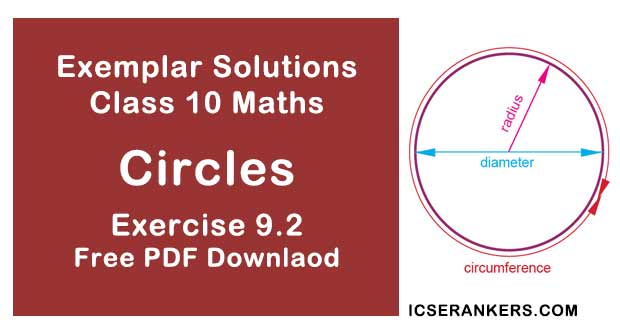Chapter Name NCERT Maths Exemplar Solutions for Chapter 9 Circles Exercise 9.2 Book Name NCERT Exemplar for Class 10 Maths Other Exercises Exercise 9.1Exercise 9.3Exercise 9.4 Related Study NCERT Solutions for Class 10 Maths

### Exercise 9.2 Solutions

Write ‘True’ or ‘False’ and justify your answer in each of the following:

1. If a chord AB subtends an angle of 60° at the centre of a circle, then angle between the tangents at A and B is also 60°.

Solution
False
Explanation:
Let us consider the given figure. In which we have a circle with centre O and AB a chord with ∠AOB = 60°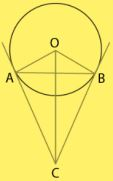As, tangent to any point on the circle is perpendicular to the radius through point of contact,
We get,
OA ⏊ AC and OB ⏊ CB
∠OBC = ∠OAC = 90° ...eq (i)
We get,
∠OBC + ∠OAC + ∠AOB + ∠ACB = 360°
90° + 90° + 60° + ∠ACB = 360°
∠ACB = 120°
Therefore, the angle between two tangents is 120°.
And, we can conclude that, the given statement is false.

2. The length of tangent from an external point on a circle is always greater than the radius of the circle.

Solution

False
Explanation:
Length of tangent from an external point P on a circle may or may not be greater than the radius of the circle.

3. The length of tangent from an external point P on a circle with centre O is always less than OP.

Solution

True
Explanation:
Consider the figure of a circle with centre O.
Let PT be a tangent drawn from external point P.
Now, Joint OT.
OT ⏊ PT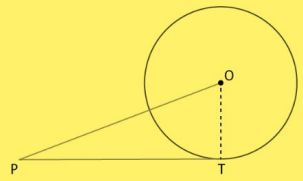We know that,
Tangent at any point on the circle is perpendicular to the radius through point of contact
Therefore, OPT is a right-angled triangle formed.
We also know that,
In a right angled triangle, hypotenuse is always greater than any of the two sides of the triangle.
So,
OP > PT or PT < OP
Hence, length of tangent from an external point P on a circle with center O is always less than
OP.

4. The angle between two tangents to a circle may be 0°.

Solution

True
Explanation:
The angle between two tangents to a circle may be 0°only when both tangent lines coincide or are parallel to each other.

5. If angle between two tangents drawn from a point P to a circle of radius a and centre O is 90°, then OP = a√2.

Solution

True.
Tangent is always perpendicular to the radius at the point of contact.
So, ∠OTP = 90
If 2 tangents are drawn from an external point, then they are equally inclined to the line segment joining the centre to that point.
Let us consider the following figure,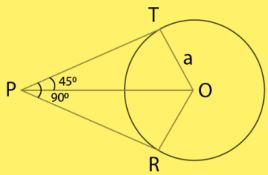From point P, two tangents are drawn.
Given,
OT = a
Also, line OP bisects the ∠RPT
∠TPO = ∠RPO = 45°
Also,
OT ⊥ TP
⇒ ∠OTP = 90°
In right angled ∆OTP,
sin 45° = OT/OP
⇒ 1/√2 = a/OP
⇒ OP = a√2

6. If angle between two tangents drawn from a point P to a circle of radius a and centre O is 60°, then OP = a√3.

Solution

False
Explanation :
From point P, two tangents are drawn.
Given,
OT = a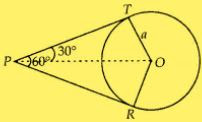Also, line OP bisects the ∠RPT.
∠TPO = ∠RPO = 30°
Also,
OT ⊥ PT
⇒ ∠OTP = 90°
In right angled ∆OTP,
sin30° = OT/OP
⇒ 1/2 =  a/OP
⇒ OP = 2a
⇒ OP = 2a

7. The tangent to the circumcircle of an isosceles triangle ABC at A, in which AB = AC, is parallel to BC.

Solution

True
Explanation:
Let EAF be tangent to the circumcircle of ∆ABC.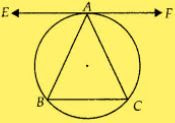To prove: EAF ॥ BC
We have, ∠EAB = ∠ACB ...(i)
[Angle between tangent and chord is equal to angle made by chord in the alternate segment]
Here, AB = AC
∠ABC = ∠ACB ...(ii)
From equation (i) and (ii), we get
∠EAB = ∠ABC
Alternate angles are equal.
EAF ॥ BC

8. If a number of circles touch a given line segment PQ at a point A, then their centres lie on the perpendicular bisector of PQ.
Solution
False
Explanation:
Given that PQ is any line segment and S1 , S2 , S3 , S4 , ..... circles touch the line segment PQ
at a point A. Let the centres of the circles S1 , S2 , S3 , S4 , ....... be C1 , C2 , C3 , C4 , .......
respectively.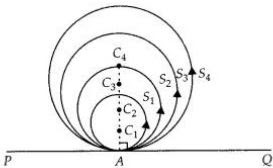To prove: Centres of the circles lie on the perpendicular bisector of PQ.
Joining each centre of the circles to the point A on the line segment PQ by line segment i.e., C1A, C2A, C3A, C4A, ...... and so on.
We know that, if we draw a line from the centre of a circle to its tangent line, then the line is always perpendicular to the tangent line. But it does not bisect the line segment PQ.

9. If a number of circles pass through the end points P and Q of a line segment PQ, then their centres lie on the perpendicular bisector of PQ.
Solution
True
Explanation:
We draw two circles with centre C1  and C2  passing through the end points P and Q of a line
segment PQ. We know that the perpendicular bisector of a chord of circle always passes
through the centre of the circle.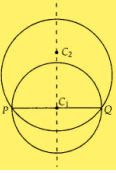Thus, perpendicular bisector of PQ passes through C1  and C2. Similarly, all the circle passing
through the end points of line segment PQ, will have their centres on the perpendicular
bisector of PQ.

10. AB is a diameter of a circle and AC is its chord such that ∠BAC = 30°. If the tangent at C intersects AB extended at D, then BC = BD.
Solution
True
To prove : BC = BD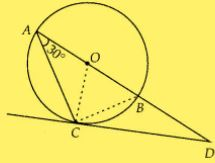Join BC and OC.
Given,
∠BAC = 30°
∠BCD = 30°
[Angle between tangent and chord is equal to angle made by chord in the alternate segment]
OC ⊥ CD and